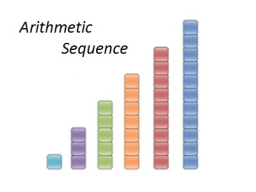# The product 9

The product of the third and second terms of the arithmetic progression is 3000. If the common difference is 10, find the first term.

a1 =  50
a2 =  -60

### Step-by-step explanation:Did you find an error or inaccuracy? Feel free to write us. Thank you!

Tips for related online calculators
Are you looking for help with calculating roots of a quadratic equation?
Do you have a linear equation or system of equations and looking for its solution? Or do you have a quadratic equation?